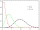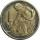# Exponential equation + reason - math problems

#### Number of problems found: 5

• Poisson distribution - daisiesThe meadow behind FLD was divided into 100 equally large parts. Subsequently, it was found that there were no daisies in ten of these parts. Estimate the total number of daisies in the meadow. Assume that daisies are randomly distributed in the meadow.
• Before yesterdayHe merchant adds a sale sign in his shop window to the showed pair of shoes in the morning: "Today by p% cheaper than yesterday. " After a while, however, he decided that the sign saying: "Today 62.5% cheaper than the day before yesterday". Determine the
• Lion or virginWe toss the coin, and every throw fits a lion or a virgin with an equal probability of 1/2. Determine how much we have to make throws that with probability 0.9 lion fell at least once.
• Compound interestCalculate time when the deposit in the bank with interest 2.5% p.a. doubles.
• When will I be a millionaire?Barry monthly send 280 euros to the bank, which he deposits bear interest of 2.1% p. A. Calculate how many months must Barry save to save 1000000 euros? Inflation, interest rate changes, or bank failures are ignored.

We apologize, but in this category are not a lot of examples.
Do you have an exciting math question or word problem that you can't solve? Ask a question or post a math problem, and we can try to solve it.

We will send a solution to your e-mail address. Solved examples are also published here. Please enter the e-mail correctly and check whether you don't have a full mailbox.

Exponential equation - math problems. Reason - math problems.